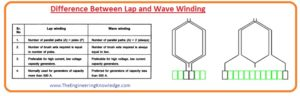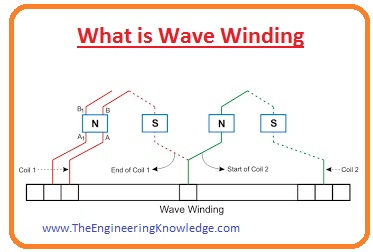Hello, friends, I hope you all are doing great. In today’s tutorial, we will discuss the Difference Between Lap and Wave Winding. The winding wounded at the armature is called armature winding. The armature winding is used to the conversion of power for generator mechanical power is transformed in the electrical power and for motor electrical energy is transformed in the mechanical power. There are 2 common types of armature windings first one is lap windings and the second one is wave winding.

The basic difference between these two windings is that in lap winding end of every coil is attached with the neighboring point and in wave winding the endpoint of winding is connected with the point at some distance. In today’s post, we will have a detailed look at both lap and wave winding with the detailed compare them to find their differences. So let’s get started with Difference Between Lap and Wave Winding.

#### Difference Between Lap and Wave Winding

Lap Winding

• In lap winding the end point of the first winding is connected with the starting point of the second winding.
• In this winding number of the parallel path is equal to the number of poles of the machines,
• Lap winding is also called Parallel Winding or Multiple Winding.
• It generated less value of EMF.
• The number of brushes needed for this winding is equal to the number of parallel paths.
• The main types of lap windings are Simplex and Duplex.
• Its efficiency is less than the wave winding.
• This winding needed Equalizer Ring.
• Due to the usage of more conductors, its cost is large.
• It used in such machines that needed less voltage and large current.

Wave Winding

• The end point of winding is connected with the commutator segment positioned at some distance.
• It has 2 number of parallel paths.
• This winding is also called Two-circuit or Series Winding.
• It generated more EMF than the lap winding.
• It needed two carbon brushes.
• The other types of wave winding are Progressive and Retrogressive wave winding.
• Its efficiency is larger than the lap winding.
• It needed an extra dummy coil.
• The price of this winding is less than the lap winding.
• It used in such machines where high voltage and less current needed.#### What is Lap Winding

• In lap winding the neighboring windings are overlapped with each other. The second end of the first winding is attached to the first end of the second coil.
• The arrangement of conductors in this winding is such that a number of parallel paths is equal to poles of machines.
• Let’s assume that there are P poles exits in the machines and Z number of the conductor so a number of parallel paths will be P and the number of the conductor in each path will be Z/P.
• The required brushes is equal to the number of parallel paths. The half brushes has a positive polarity and half have a negative polarity.
• There are 2 main types of lap windings.Simplex Lap Winding

• This winding has a number of parallel paths equal to the number of poles.

Duplex Lap Winding

• In this windings number of the parallel paths is 2 times the poles of machines.

#### What is Wave Winding

• The one terminal of the coil is attached with the initial point of the second winding that has similar polarity like first winding.
• As the arrangement of winding is like a wave so it is known as wave winding.
• The conductor of wave winding is divided in the 2 parallel paths and every path consists of Z/2 conductors/
• The brushes used are 2 and the number of paths is also 2.#### Comparison between Lap and Wave Winding

• The configuration of lap winding is that the first winding is lap back to the neighboring winding while in wave winding windings are attached in a wave shape.
• The end point of the first winding is attached with the next commutator point and in wave windings first, the winding end is located at some distance.
• There are 2 parallel path exits in the wave winding and in lap same path exits as a number of poles.
• The lap winding is known as parallel winding and wave winding is called series winding.
• The wave winding has a large emf than the lap.
• To get better commutation equalizers are used in lap winding and for mechanical balance dummy winding is used.
• The brushes used in lap winding are equal to the number of parallel paths while in wave winding 2 brushes are used.
• Wave winding gives large efficiency than the lap.
• The types of lap windings are simplex and duplex while types of waves are progressive and retrogressive.
• The wave winding is less expensive than the lap.
• In less voltage applications lap winding is preferred and in high voltage wave winding is used.

So friends that is a detailed post about the difference between lap and wave winding. If you have any query ask in the comments. Thanks for reading. Have a good day.